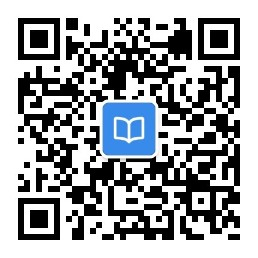#### 有 VBA 语句：If x=1 then y=1，下列叙说中正确的是

• A.x=1 和 y=1 均为赋值语句
• B.x=l 和 y=1 均为关系表达式
• C.x=1 为关系表达式，y=1 为赋值语句
• D.x=1 为赋值语句，y=1 为关系表达式2023年计算机等级考试题库